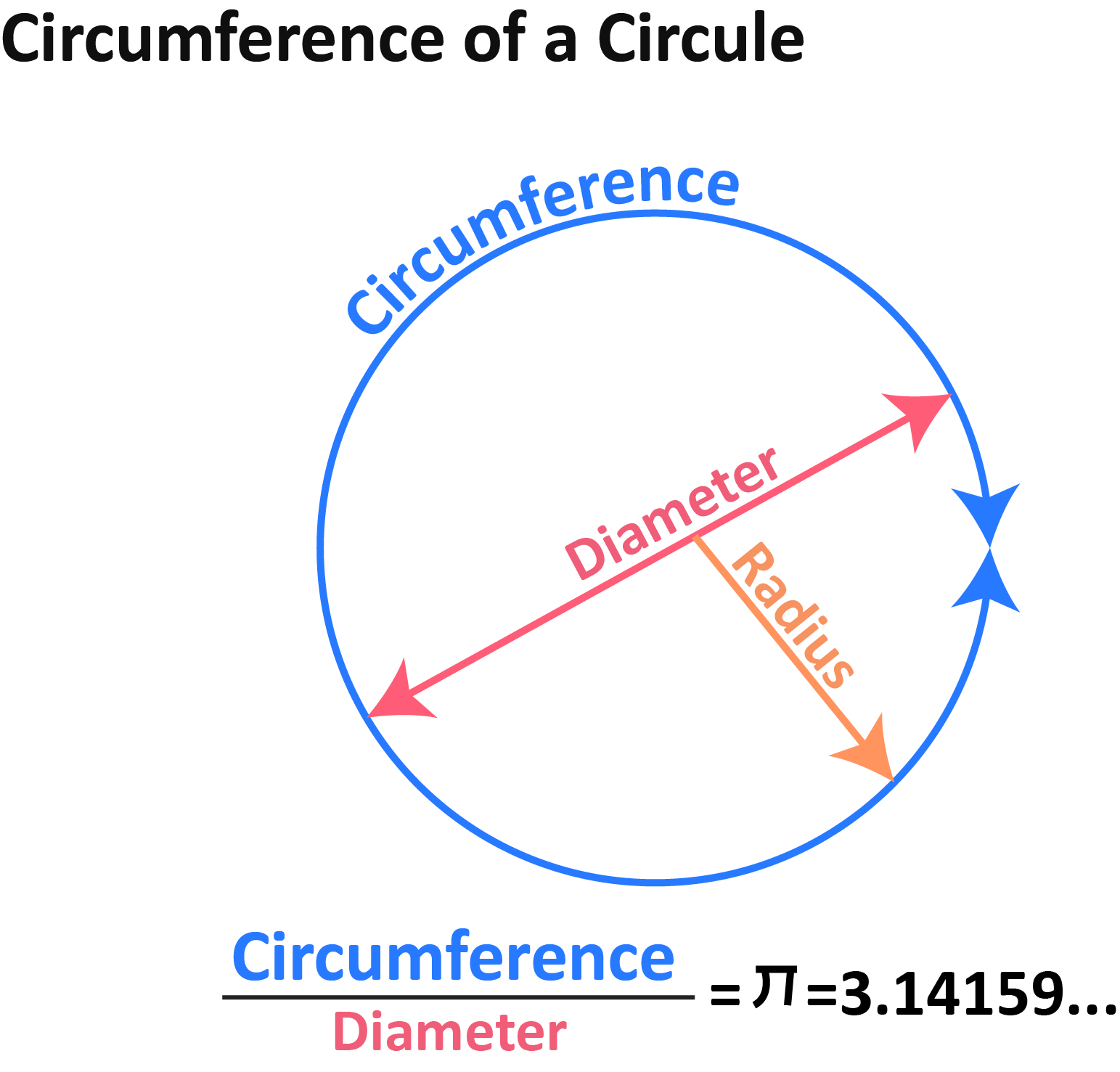Uh Oh! It seems you’re using an Ad blocker!

We always struggled to serve you with the best online calculations, thus, there's a humble request to either disable the AD blocker or go with premium plans to use the AD-Free version for calculators.

Or# Circumference to Diameter Calculator

Enter the diameter or circumference in the tool to find the relative measurements of circle

Circumference :

Diameter :

Table of Content

Get the Widget!

Add this calculator to your site and lets users to perform easy calculations.

Feedback

How easy was it to use our calculator? Did you face any problem, tell us!

Use the circumference to diameter calculator for precise and accurate conversion of diameter from circumference and vice versa. Get complete steps involved to get the diameter from the circumference with utmost accuracy.

## Circle:

A circle is round and symmetrical and each point is equidistant from its center. A circle has several properties which include the circumference and diameter.

## Circumference:

It is the perimeter of a circle and the measure of the boundary or the area of circular edges. Circumference is the total length of the outer edges of the circle.

## Diameter:

It is a straight line that passes through the center of the circle and extends from one edge. The diameter connects two points of the circumference with each other and it represents the width of the circular object. The circumference to diameter calculator convert circumference to diameter and vice versa.

## How to Find Diameter from Circumference?

The diameter of a circle formula from circumference is:

d = C/π

The circumference of the circle is:C = πd

Where:

d = diameter

C = Circumference

π = ratio of a circle’s circumference to its diameter

The diameter to circumference calculator provides an easy procedure to calculate diameter from circumference.

### Practical Example 1:

Let’s suppose the diameter of a circle is 10 cm, then what is its circumference?

Given:

D = 10 cm

C =?

Solution:

C =  πd

C = (3.14)(10)

C = 31.416 cm

The circumference of a circle to diameter conversion in other units:

 mm m km in ft yd mi 314.159 0.314 0.000314 12.37 1.031 0.34357 0.000195

You can check all the conversion with our online free circumference to diameter calculator to make more measurements precise and accurate.

### Practical Example 2:

Let’s suppose the circumference of the circle is 60, then calculate diameter from circumference.

Given:

C = 60 cm

d = ?

Sol:

d = C/π

d = 60/π

d = 19.099 cm

Diameter of circle from circumference conversions in other units:

 mm m km in ft yd mi 190.986 0.191 0.000191 7.52 0.627 0.20886 0.000119

## Working of Circumference to Diameter Calculator:

Our calculator does conversion from diameter to circumference in no time if provided with the following inputs:

Input:

• Enter the circumference and choose the unit
• Tap the Calculate

Output:

• Diameter of circle
• Other conversion units

## FAQs:

### What is the Circumference of a Circle with a Radius 24 inches?

Circumference = 2×π×r

C = 2×3.14×24

C = 150.72 inches

### What are the Steps Involved in Finding the Circumference of a Circle if its Area is Given?

• Find the radius using the area of the circle formula (A = πr2).
• Now, substitute the radius value in the circumference formula (C = 2πr) to get the answer.

### Which Formula is Used to find the Perimeter of a circle if its Area is Given?

If the area of a circle is given, then the formula to calculate the perimeter or circumference of a circle is given by: C = 2√(πA) units.

### What is the Circumference of a Circle in Terms of π, if its Diameter is 7 cm?

As we know, the Circumference of a circle if its diameter is given = π × d units.

Substituting d = 7 cm in the formula, we get

C = π × 7 = 7π units.

## References:

From the source of Wikipedia: Circumference, Circle